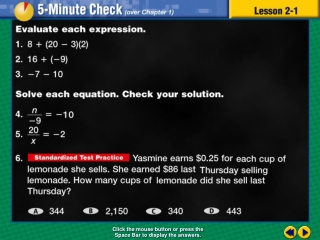Download PresentationTransparency 1# Transparency 1 - PowerPoint PPT Presentation

Download Presentation##### Transparency 1

Download Policy: Content on the Website is provided to you AS IS for your information and personal use and may not be sold / licensed / shared on other websites without getting consent from its author. While downloading, if for some reason you are not able to download a presentation, the publisher may have deleted the file from their server.

- - - - - - - - - - - - - - - - - - - - - - - - - - - E N D - - - - - - - - - - - - - - - - - - - - - - - - - - -
##### Presentation Transcript

1. Transparency 1 Click the mouse button or press the Space Bar to display the answers.

2. Splash Screen

3. Example 1-5c Objective Express rational numbers as decimals and decimals as fractions

4. Example 1-5c Vocabulary Rational number Any number that can be expressed in the form a/b, where a and b are integers and b ≠ 0 All integers, fractions, and mixed numbers are rational numbers

5. Example 1-5c Vocabulary Terminating decimal A decimal when the division ends, or terminates, and the remainder is 0 .625

6. Example 1-5c Vocabulary Repeating decimal A decimal where it is not possible to write all the digits without a remainder .666. . . Would be written .6

7. Example 1-5c Vocabulary Bar notation A bar placed above the repeating part of a decimal 8.6363636363. . . 8.63

8. Lesson 1 Contents Example 1Write a Fraction as a Decimal Example 2Write a Mixed Number as a Decimal Example 3Round a Repeating Decimal Example 4Write a Terminating Decimal as a Fraction Example 5Write a Repeating Decimal as a Fraction

9. Write as a decimal. ENTER 3 16 0.1875 Example 1-1a Write problem Use the calculator Put numerator in calculator which is 3 Push  Answer: 0.1875 Put denominator in calculator which is 16 Push = 1/5

10. Write as a decimal. Example 1-1c Answer:0.0625 1/5

11. Write as a decimal. ENTER 2 11 Example 1-2a Write problem Use the calculator Write the whole number which is 3 and then a decimal Put numerator in calculator which is 2 Push  3. Put denominator in calculator which is 11 Push = 2/5

12. Write as a decimal. ENTER 2 11 0.1818181818 Example 1-2a Notice: a zero is in the one’s place on the calculator The zero has no value as a whole number so drop it There is a repeating number which is 18 3. 3.18 Put 18 to the right of the decimal Put a bar notation over the 18 since it is repeating Answer: 3.18 2/5

13. Write as a decimal. Example 1-2c Answer:5.1 2/5

14. Example 1-3a AGRICULTUREA Florida farmer lost the fruit on 8 of 15 orange trees because of unexpected freezing temperatures. Find the fraction of the orange trees that did not produce fruit, then write it as a decimal Write problem 8 . 15 Remember: a fraction is a part over the whole Part: 8 that did not produce fruit Whole: All the orange trees 3/5

15. ENTER 8 15 Example 1-3a Now write as decimal using the calculator 8 . Put numerator in calculator which is 8 15 Push  Put denominator in calculator which is 15 0.53333 . . . Push = 3 is repeating so write 0.53 Answer: 0.53 Put a bar notation over the repeating 3 3/5

16. Example 1-3b SCHOOLIn Mrs. Hinote’s eighth grade science class, 4 out of 22 students did not turn in their homework. Find the fraction of the students who did not turn in their homework, then write it as a decimal rounding to the nearest hundredth. Answer:0.18 3/5

17. Example 1-4a Write problem Use the calculator Write 0.32 as a fraction. Put number in calculator as it is read 0.32 0.32 Push the 2nd key 2nd 0.32 Push the This key converts fractions to decimals and decimals to fractions 4/5

18. Example 1-4b Write 0.16 as a fraction. Answer: 4/5

19. ALGEBRAWrite as a mixed number. Example 1-5a Write problem Use the calculator Remember the bar notation over the 7 means the 7 is being repeated Put number in calculator 2.7 and repeat the 7 several time 2.77777 2nd Push the 2nd key Push the Answer: 5/5

20. ALGEBRAWrite as a mixed number. Example 1-5c * Answer: 5/5

21. End of Lesson 1 Assignment